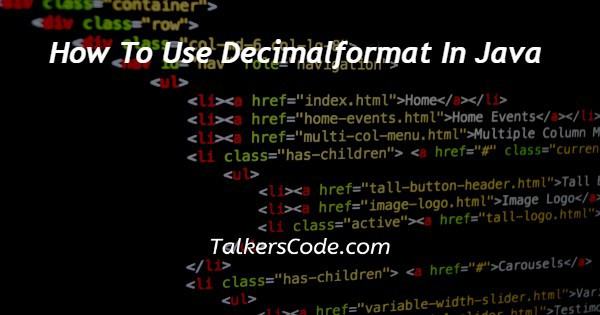# How To Use Decimalformat In Java

Last Updated : Aug 9, 2023In this article we will show you the solution of how to use decimalformat in java, with the Java.text.DecimalFormat class, numbers can be formatted according to the formatting pattern that you specify.

The purpose of this text is to explain how to use the DecimalFormat class to format different types of numbers.

As if it were going to be created using the new pattern, the applyPattern() method applies a pattern to the DecimalFormat object.

Similar to applyPattern(), applyLocalizedPattern() interprets the characters in the pattern in accordance with the Locale the DecimalFormat was created with.

As a consequence, the characters indicating the decimal separator must appear. Instead of just using the standard marking characters in the pattern, the patterns are now interpreted based on the characters used for that locale.

We will now discuss the idea of how to use decimalformat in java with an example.

## Step By Step Guide On How To Use Decimalformat In Java :-

```package javaDecimalFormat;
import java.text.DecimalFormat;
import java.text.DecimalFormatSymbols;
import java.text.NumberFormat;
import java.text.ParseException;
import java.util.Currency;
import java.util.Locale;
public class TC{
public static void main(String[] args) throws ParseException {
DecimalFormatSymbols decimalSymbol = new DecimalFormatSymbols(Locale.INDIA);
decimalSymbol.setDecimalSeparator('-');
decimalSymbol.setGroupingSeparator('!');
decimalSymbol.setExponentSeparator("I");
decimalSymbol.setInfinity("infinity");
decimalSymbol.setDigit('1');
decimalSymbol.setCurrency(Currency.getInstance(Locale.INDIA_AMERICA));
String pattern = "###,##,###";
DecimalFormat decimalFormat = new DecimalFormat(pattern, decimalSymbol);
decimalFormat.setGroupingSize(4);
String number = decimalFormat.format(342128.234221);
System.out.println("The currency is : " + decimalFormat.getCurrency());
System.out.println("the number is " + number);
}
}```
1. The first step is to import the Java packages and classes needed, such as DecimalFormat, DecimalFormatSymbols, NumberFormat, ParseException, and Currency.
2. In order for the program to function, we must define a main function.
3. As part of creating DecimalFormatSymbols, we assign the Locale.INDIA constant to set the default formatting symbols for Indian locales.
4. Our next step was to set a few custom formatting symbols on this instance, such as the decimal separator as '-' and the grouping separator as '!'.
5. As well as custom values for exponent separators ("I"), infinity symbols ("infinity"), and digit symbols ('1'), we also set custom values for other formatting symbols.
6. The next step is to define a formatting pattern for numbers using the "###,##,###" format. This format specifies that each group of three digits should be separated by commas.
7. After this, we create a new instance of DecimalFormat and assign the custom pattern and formatting symbols as required.
8. In this number format, we group decimals by four instead of three, which means they are grouped every four digits.
9. Our next step is to format a test number (342128.234221) according to this format and save the result to the String variable number.
10. At the end of the process, we print the formatted number and the currency symbol associated with the number format (using the getCurrency() method).

## Conclusion :-

As a result, we have successfully learned how to use decimalformat in java with an example.

Java DecimalFormat is a powerful tool that allows users to create desirable formats by setting everything accordingly; separators like decimal grouping exponential can be represented with the user's choice of symbol, as well as rounding off data types to decimal points of choice for double and float.

I hope this article on how to use decimalformat in java helps you and the steps and method mentioned above are easy to follow and implement.